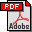In-place calculation of minimum-redundacy codes Authors: Alistair Moffat and Jyrki Katajainen Published in: Proceedings of the 4th Workshop on Algorithms and Data Structures, Lecture Notes in Computer Science 955, Springer-Verlag (1995), 393-402 Full text:PDF (639 kB) DOI: 10.1007/3-540-60220-8_79 Copyright: © Springer-Verlag Abstract: The optimal prefix-free code problem is to determine, for a given array p = [pi | i ∈ {1… n}] of n weights, an integer array l = [li | i ∈ {1… n}] of n codeword lengths such that ∑i=1n 2−li ≤ 1 and ∑i=1n pi li is minimized. Huffman’s famous greedy algorithm solves this problem in O(n logn) time, if p is unsorted; and can be implemented to execute in O(n) time, if the input array p is sorted. Here we consider the space requirements of the greedy method. We show that if p is sorted then it is possible to calculate the array l in-place, with li overwriting pi, in O(n) time and using O(1) additional space. The new implementation leads directly to an O(n logn)-time and n+O(1) words of extra space implementation for the case when p is not sorted. The proposed method is simple to implement and executes quickly. Keywords. Prefix-free code, Huffman code, in-place algorithm, data compression. BibLATEX: ```@inproceedings{MK1995bC, author = {Alistair Moffat and Jyrki Katajainen}, title = {In-place calculation of minimum-redundacy codes}, booktitle = {Proceedings of the 4th Workshop on Algorithms and Data Structures}, series = {Lecture Notes in Computer Science}, volume = {955}, publisher = {Springer-Verlag}, year = {1995}, pages = {393--402}, } ```